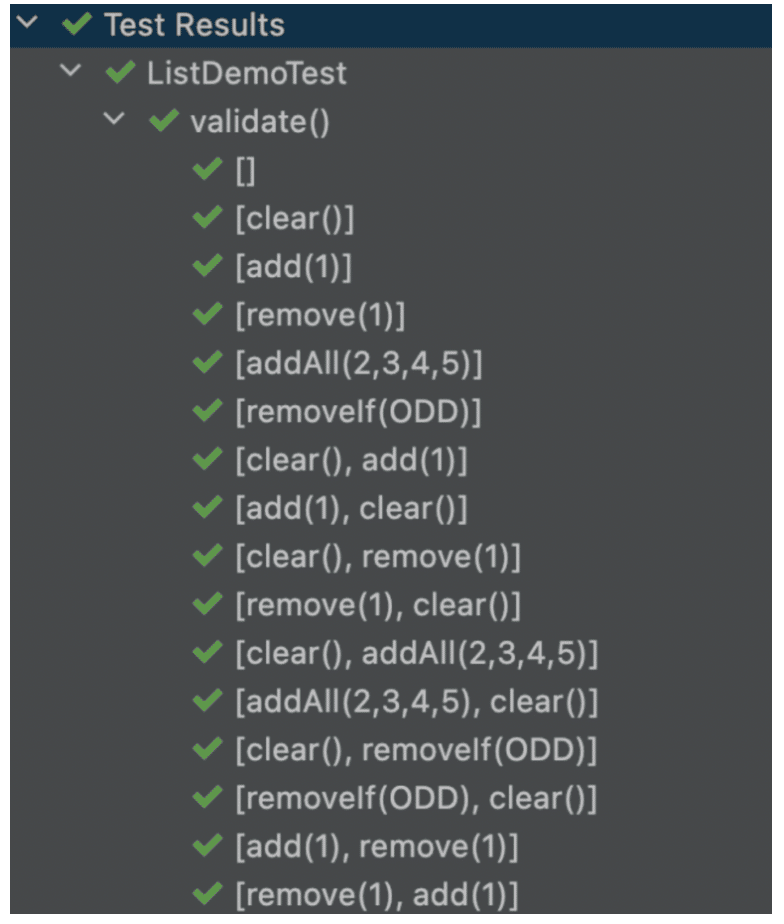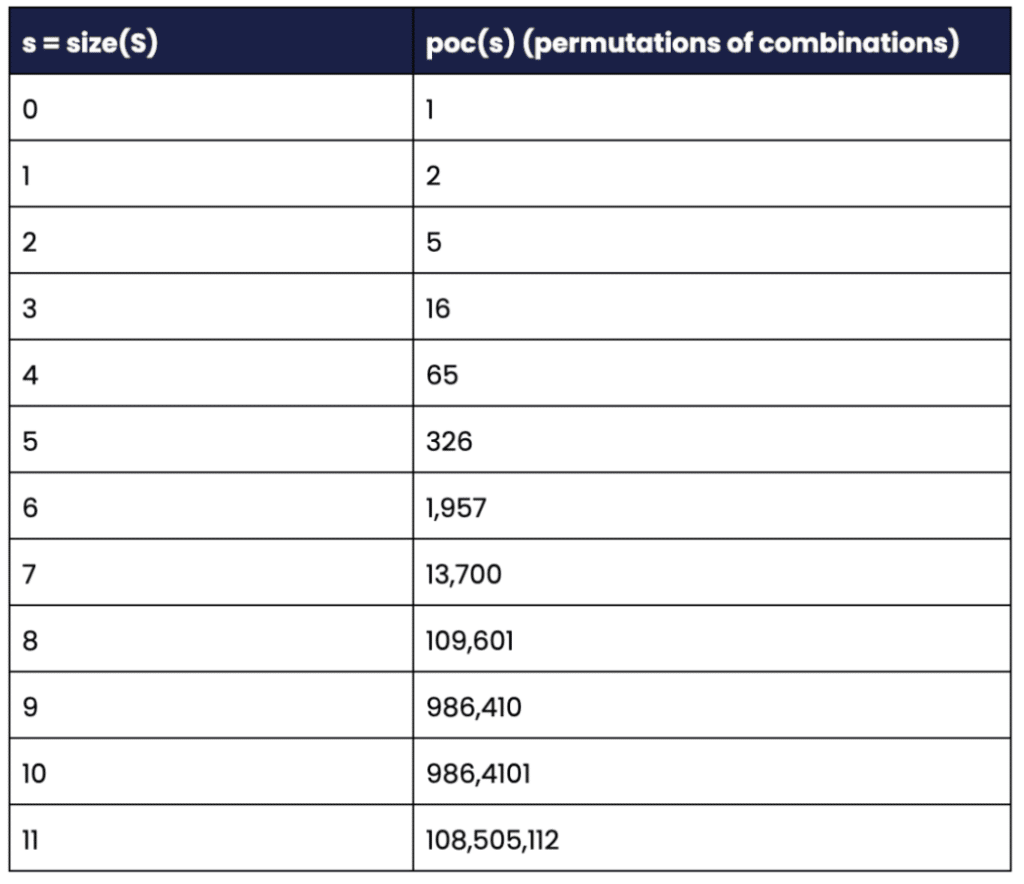# Exhaustive JUnit5 Testing with Combinations, Permutations and Products

March 08, 2023

## Author(s)

•Per Minborg is an inventor and developer with more than 20 years of Java coding experience. He is a frequent contributor to open-source projects, an Oracle Groundbreaker Ambassador, an Oracle ... Learn more

Unit testing constitutes an integral part of the process of providing high-quality software.

But, how can one write tests covering all variants of several operations?

### Test Support Libraries

There are many libraries that make testing better in different aspects. Here are some of them:

#### Agitar One

Agitator automatically creates dynamic test cases, synthesizes sets of input data, and analyzes the results.

#### Chaos Monkey

Chaos Monkey is responsible for randomly terminating instances in production to ensure that engineers implement their services to be resilient to instance failures.

#### Chronicle Test Framework

This library provides support classes for writing JUnit tests. It supports both JUnit4 and JUnit5. Tools like permutations, combinations and products can be leveraged to create exhaustive tests.

Agitar One can automatically identify and generate tests whereas Chronicle Test Framework provides programmatic support for writing your own tests.

### Objective

Suppose we have written a custom implementation of java.util.List called MyList and that we want to test the implementation against a reference implementation such as ArrayList.

Further, assume that we have a number of operations O1, O2, …, On. that we can apply to these objects.

The question now is: how can we write tests assuring that the two objects provide the same result regardless of how the operations are applied?

### Combinations, Permutations and Products

Before we continue, we remind ourselves of what combinations, permutations, and products are from a mathematical perspective. Assume we have a set X = {A, B, C} then;

The combinations of X are:

```[]
[A]
[B]
[C]
[A, B]
[A, C]
[B, C]
[A, B, C]```

The permutations of X are:

```[A, B, C]
[A, C, B]
[B, A, C]
[B, C, A]
[C, A, B]
[C, B, A]```

The permutations of all combinations of X are:

```[]
[A]
[B]
[C]
[A, B]
[B, A]
[A, C]
[C, A]
[B, C]
[C, B]
[A, B, C]
[A, C, B]
[B, A, C]
[B, C, A]
[C, A, B]
[C, B, A]```

Where [] denotes a sequence of no elements. As can be seen from the sequences above, the number of variants will increase very rapidly as the number of set members increases.

This puts a practical limit on the exhaustiveness of the test one can write.

Another concept (not exemplified in this article) is “Products” (aka Cartesian Products). Suppose we have a sequence s1 = [A, B] of one type and another sequence s2 = [1, 2] of another type then;

The product of s1 and s2 is:

```[A, 1]
[A, 2]
[B, 1]
[B, 2]```

Products have many similarities with database “join” operations and relate to nested loops.

### The Test Framework

In this test, we will be using open-source Chronicle-Test-Framework that supports the aforementioned combination, permutation, and product features. Here is an example:

```// Prints: [], [A], [B], [C], [A, B], [B, C], … (8 sequences)
Combination.of("A", "B", "C")
.forEach(System.out::println)```

This prints all the combinations of {A, B, C} the result being the same as in the previous chapter. In the same way, the following example will print out all the permutations of {A, B, C}:

```// Prints: [A, B, C], [A, C, B], [B, A, C], … (6 sequences)
Permutation.of("A", "B", "C")
.forEach(System.out::println)```

In this following example, we combine the capabilities of combinations and permutations:

```// Prints: [], [A], [B], [C], [A, B], [B, A], …(16 sequences)
Combination.of("A", "B", "C")
.flatMap(Permutation::of)
.forEach(System.out::println)```

The methods above produce a Stream of Collection elements allowing easy adaptations to other frameworks such as JUnit5.

When it comes to products, things work slightly differently. Here is an example:

```final List<String> strings = Arrays.asList("A", "B");
final List<Integer> integers = Arrays.asList(1, 2);

Product.of(strings, integers)
.forEach(System.out::println);```

This will print:

```Product2Impl{first=A, second=1}
Product2Impl{first=A, second=2}
Product2Impl{first=B, second=1}
Product2Impl{first=B, second=2}```

If a more recent Java version is used, the following scheme would typically be used instead:

```record StringInteger(String string, Integer integer){}
Product.of(strings, integers, StringInteger::new)
.forEach(System.out::println);```

This will produce:

```StringInteger[string=A, integer=1]
StringInteger[string=A, integer=2]
StringInteger[string=B, integer=1]
StringInteger[string=B, integer=2]```

In my opinion, the above is better than the default Product2Impl tuple because the record is a “nominal tuple” where the names and types of the state elements are declared in the record header compared to the Product2Impl which relies on generic types and “first” and “second” as names.

### The Objective

Suppose that we want to make sure two java.lang.List implementations behave the same way for a number of mutating operations. Here, lists contain Integer values (ie implements List).

We start by identifying the mutating operations to use. One (arbitrary) suggestion is to use the following operations:

```list.clear()
list.remove((Integer) 1) // Will remove the object 1 and not @index 1
list.removeIf(ODD)
Where Predicate<Integer> ODD = v -> v % 2 == 1.```

As the reader might suspect, the List types are going to be tested against each other with all the possible sequences of operations. But, how can this be achieved?

### The Solution

JUnit5 provides a @TestFactory annotation and DynamicTest objects, allowing a test factory to return a Stream of DynamicTest instances.

This is something that we can leverage, as shown below:

```private static final Collection<NamedConsumer<List<Integer>>> OPERATIONS =
Arrays.asList(
NamedConsumer.of(List::clear, "clear()"),
NamedConsumer.of(list -> list.remove((Integer) 1), "remove(1)"),
NamedConsumer.of(list -> list.addAll(Arrays.asList(2, 3, 4, 5)),
NamedConsumer.of(list -> list.removeIf(ODD), "removeIf(ODD)")
);

@TestFactory
Stream<DynamicTest> validate() {
return DynamicTest.stream(Combination.of(OPERATIONS)
.flatMap(Permutation::of),
Object::toString,
operations -> {
List<Integer> first = new ArrayList<>();
operations.forEach(op -> {
op.accept(first);
op.accept(second);
});
assertEquals(first, second);
});
}```

This is the entire solution, and when run under IntelliJ (or other similar tools), the following tests will be performed (only the first 16 of the 326 tests are shown for brevity):Image 1, Shows the first 16 test runs of the 326 tests.

The reason NamedConumer is used over the standard java.util.function.Consumer is that it allows real names to be shown rather than some cryptic lambda reference like ListDemoTest\$\$Lambda\$350/0x0000000800ca9a88@3d8314f0.

It certainly looks better in the output and provides much better debug capabilities.

### Expanding the Concept

Suppose we have a more significant number of List implementations we’d like to test, such as:

```ArrayList
CopyOnWriteArrayList
Stack
Vector```

To expand the concept, we begin by creating a collection of List constructors:

```private static final Collection<Supplier<List<Integer>>> CONSTRUCTORS =
Arrays.asList(
ArrayList::new,
CopyOnWriteArrayList::new,
Stack::new,
Vector::new);```

The reason for working with constructors rather than instances is that we need to be able to create new List implementations for each dynamic test.

Now, we only need to do minor modifications to the previous test:

```@TestFactory
Stream<DynamicTest> validateMany() {
return DynamicTest.stream(Combination.of(OPERATIONS)
.flatMap(Permutation::of),
Object::toString,
operations -> {

// Create a fresh list of List implementations
List<List<Integer>> lists = CONSTRUCTORS.stream()
.map(Supplier::get)
.collect(Collectors.toList());

// For each operation, apply the operation on each list
operations.forEach(lists::forEach);

// Test the lists pairwise
Combination.of(lists)

// Filter out only combinations with two lists
.filter(set -> set.size() == 2)

// Convert the Set to a List for easy access below
.map(ArrayList::new)

// Assert the pair equals
.forEach(pair -> assertEquals(pair.get(0), pair.get(1)))
});
}```

Strictly, this is a bit of cheating as a single dynamic test contains several subtests for a plurality of List pairs.

However, It would be reasonably easy to modify the code above to flatMap() in the various assertions under separate dynamic tests. This is left to the reader as an exercise.

### A Final Warning

Combinations, permutations, and products are potent tools that can increase test coverage, but they can also increase the time it takes to run all tests as the number of sequences explodes even with only a moderate increase in the number of input variants.

For example, here is a list of the number of permutations of all combinations for some given sets S of size s:Table 1, shows the number of permutations of combinations for some set sizes ‘s’.

More generally, it can be shown that: poc(s) = poc(s – 1) * s + 1.

If in doubt, the number of combinations can be checked using the .count() stream operation which will return the number of sequences returned as shown below:

```long poc6 = Combination.of(1, 2, 3, 4, 5, 6)
.flatMap(Permutation::of)
.count();```

The stream above will, unsurprisingly, return 1,957 as there are six input elements.

Combinatorics can make a huge impact. However, make sure to keep the number of dynamic tests under control, or else test time might increase significantly.

Libraries like Chronicle Queue and Chronicle Map make use of the Chronicle-Test-Framework to improve testing. Here is an example from Chronicle Map where five Map operations are exhaustively tested providing 326 dynamic tests in just a few lines of code.

Resources

Topics:

## Related Articles

• Challenges when Developing a GUI for FIX

In this article, we explore the challenges in developing a Graphical User Interface (GUI) for Financial Information Exchange (FIX) data.December 21, 2022
• Chronicle Queue Storing 1TB in Virtual Memory on a 128GB Machine

Chronicle Queue and Chronicle Map allows you to have a persisted store which can be embedded into multiple JVMs on the same server.September 27, 2022
• Creating Terabyte Sized Queues with Low-Latency

Learn how to create huge persisted queues while retaining predictable and consistent low latency using open-source Chronicle Queue!November 02, 2022

## Author(s)

•Per Minborg is an inventor and developer with more than 20 years of Java coding experience. He is a frequent contributor to open-source projects, an Oracle Groundbreaker Ambassador, an Oracle ... Learn more